# Splay入门解析【保证让你看不懂（滑稽）】

$$\text{Splay}$$如果需要严格的证明均摊复杂度参考势能分析。

QwQ......

BST真是神奇的东西。。。

orzCJ老爷，各种树都会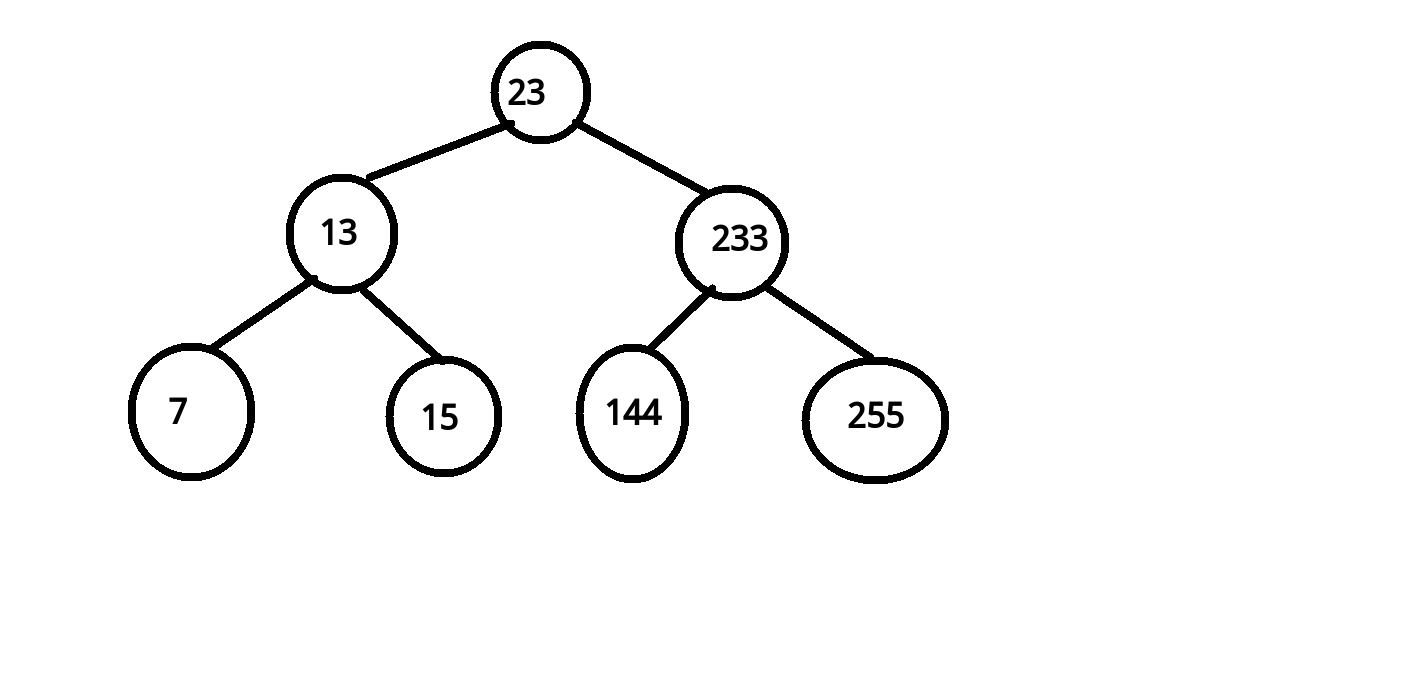X是Y的左儿子，所以X < Y
Y是Z的左儿子，所以Y < Z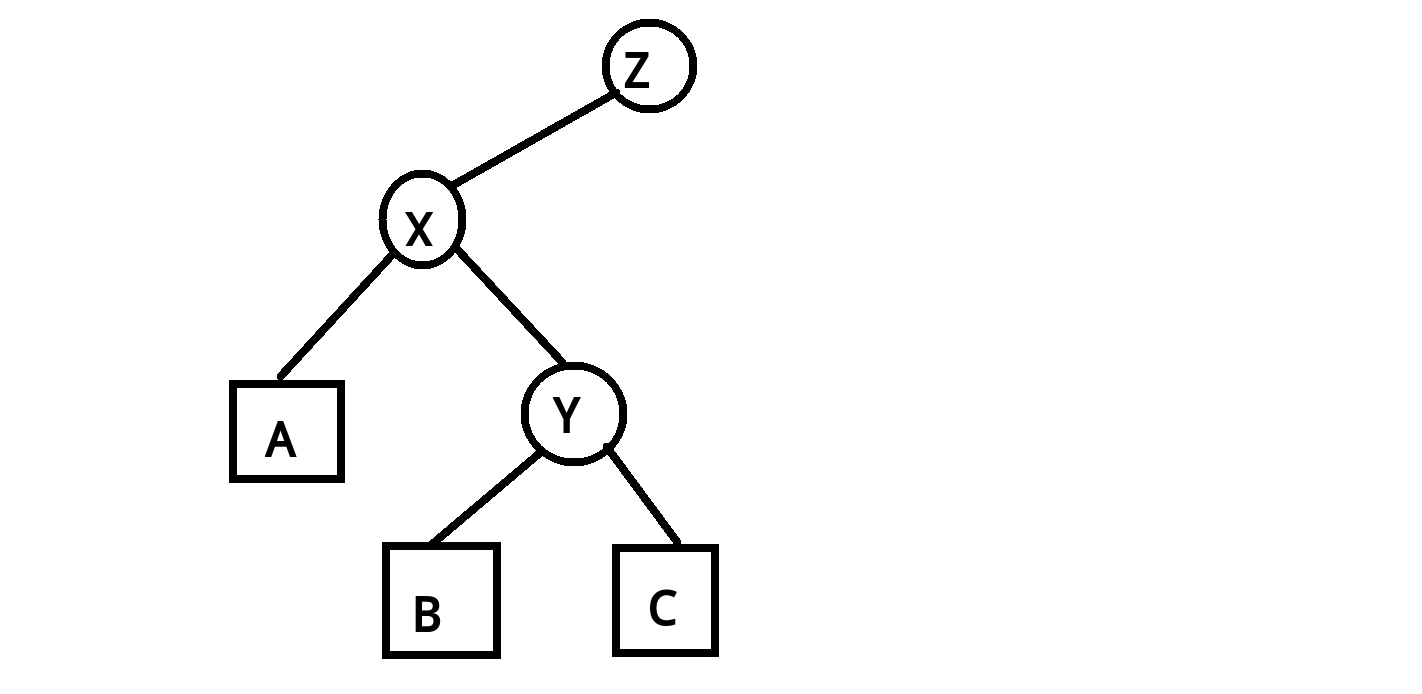A < X < B < Y < C < Z

A < X < B < Y < C < Z

X是Y的左儿子，A是X的左儿子，旋转完之后，A还是X的左儿子

1.X变到原来Y的位置
2.Y变成了 X原来在Y的 相对的那个儿子
3.Y的非X的儿子不变 X的 X原来在Y的 那个儿子不变
4.X的 X原来在Y的 相对的 那个儿子 变成了 Y原来是X的那个儿子

void rotate(int x)//X是要旋转的节点
{
int y=t[x].ff;//X的父亲
int z=t[y].ff;//X的祖父
int k=t[y].ch==x;//X是Y的哪一个儿子 0是左儿子 1是右儿子
t[z].ch[t[z].ch==y]=x;//Z的原来的Y的位置变为X
t[x].ff=z;//X的父亲变成Z
t[y].ch[k]=t[x].ch[k^1];//X的与X原来在Y的相对的那个儿子变成Y的儿子
t[t[x].ch[k^1]].ff=y;//更新父节点
t[x].ch[k^1]=y;//X的 与X原来相对位置的儿子变成 Y
t[y].ff=x;//更新父节点
}


t[y].ch是y的右儿子，如果x是右儿子，那么这个式子是1，否则是0，也正好对应着左右儿子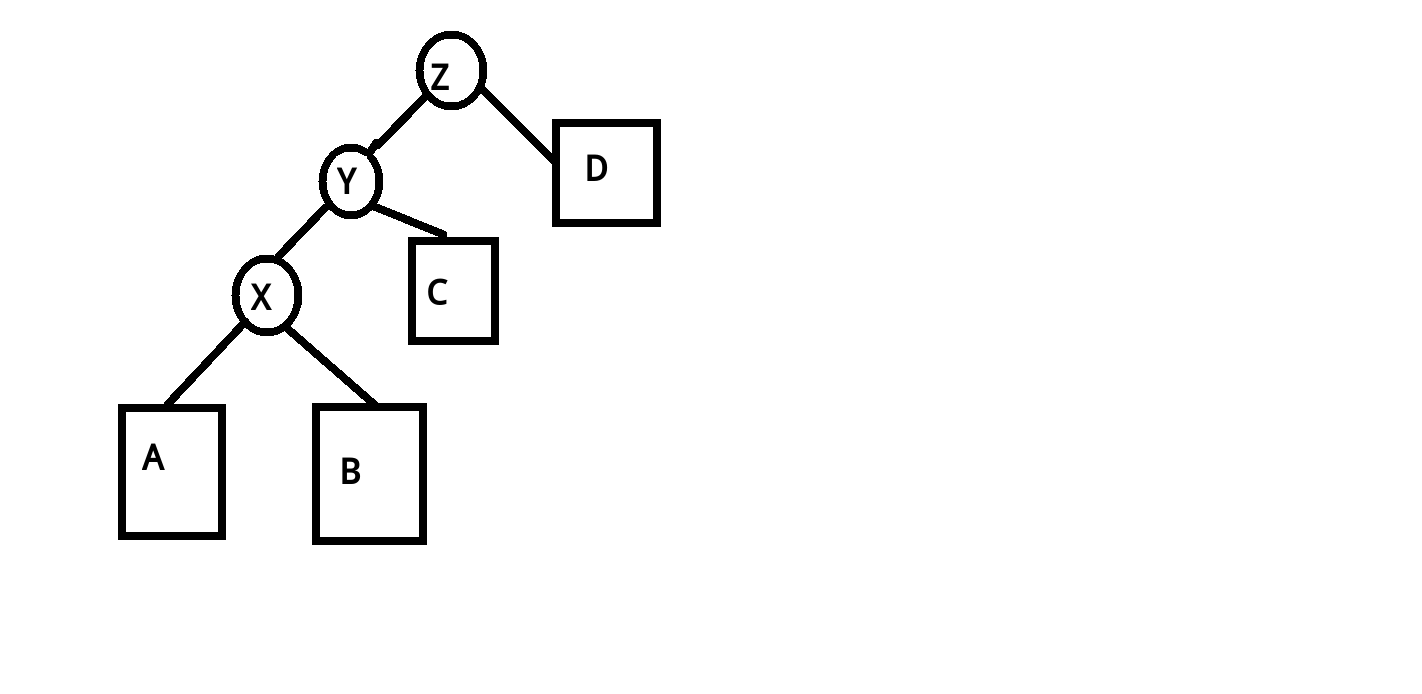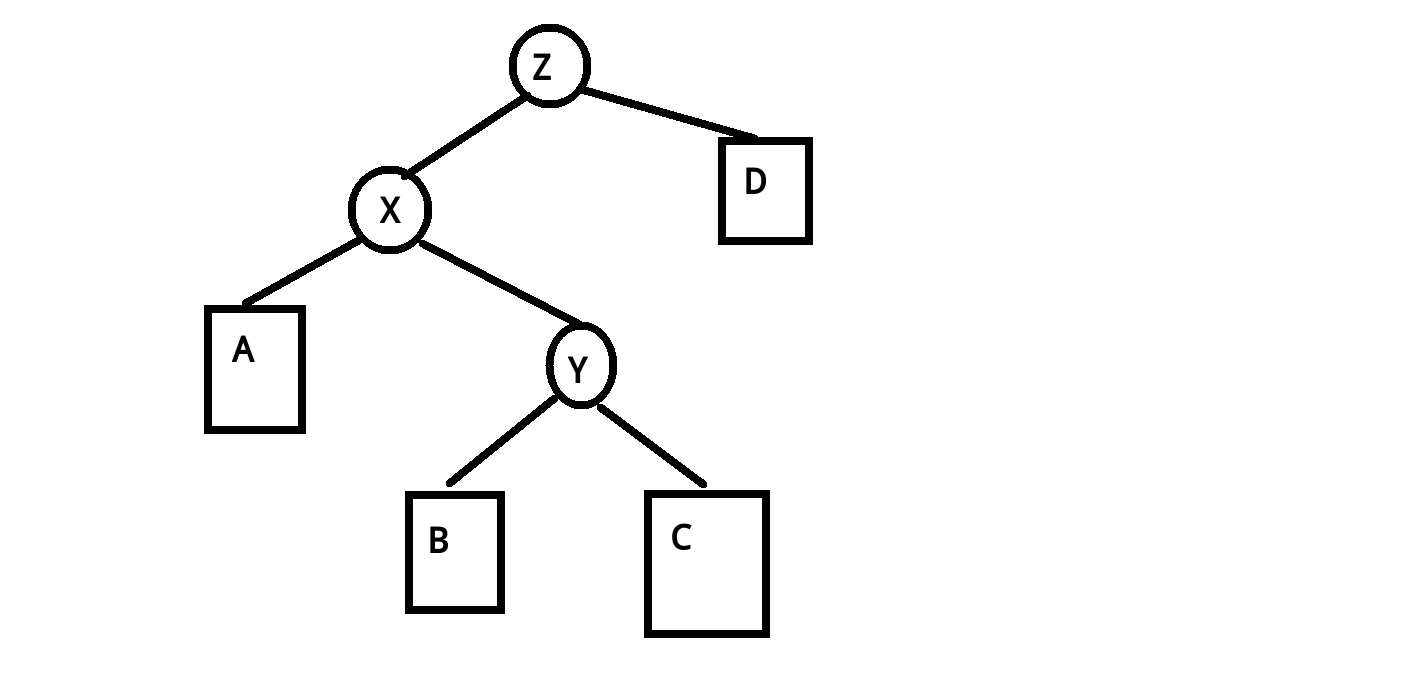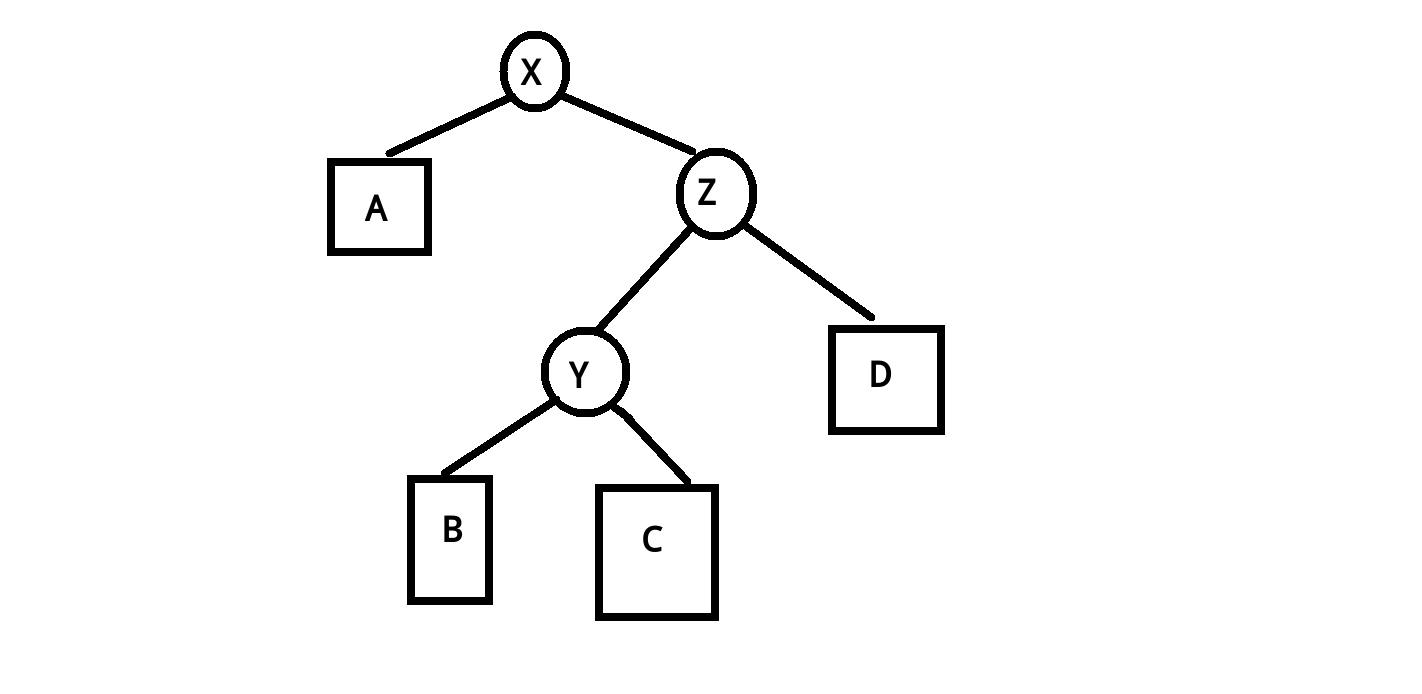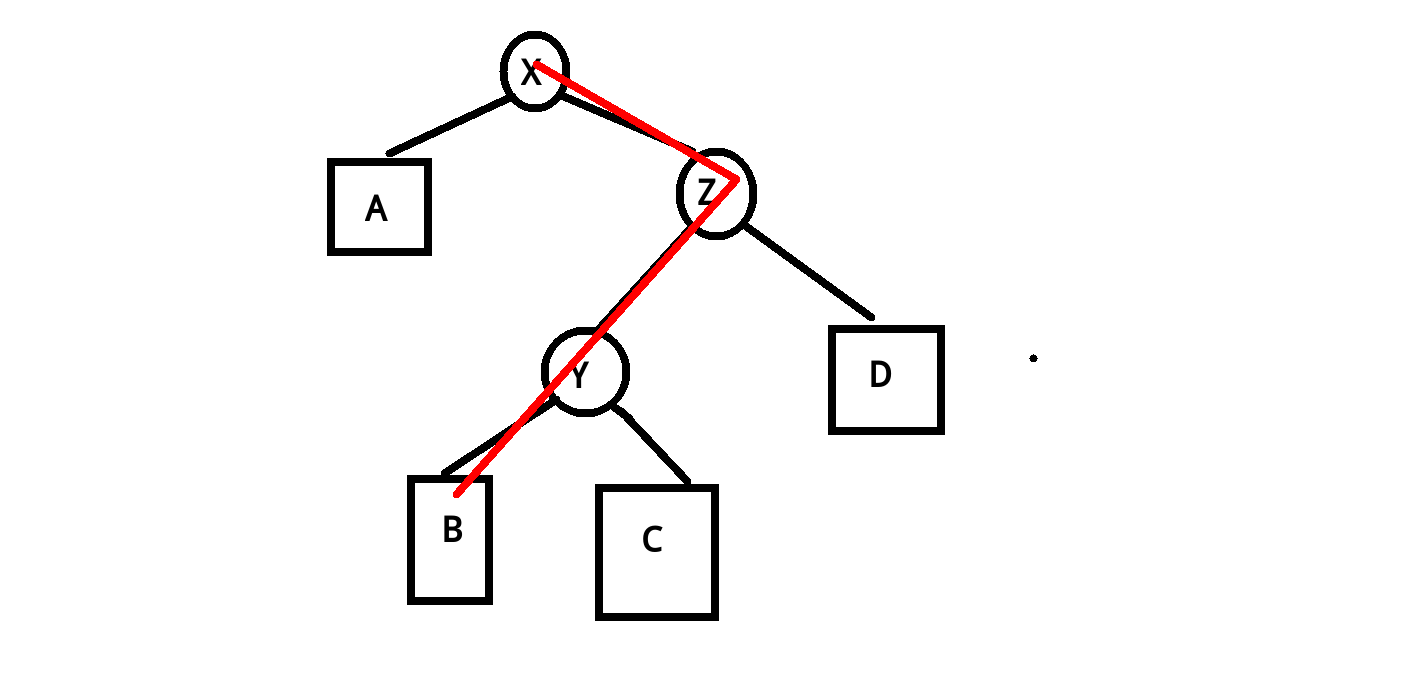void splay(int x,int goal)//将x旋转为goal的儿子，如果goal是0则旋转到根
{
while(t[x].ff!=goal)//一直旋转到x成为goal的儿子
{
int y=t[x].ff,z=t[y].ff;//父节点祖父节点
if(z!=goal)//如果Y不是根节点，则分为上面两类来旋转
(t[z].ch==y)^(t[y].ch==x)?rotate(x):rotate(y);
//这就是之前对于x和y是哪个儿子的讨论
rotate(x);//无论怎么样最后的一个操作都是旋转x
}
if(goal==0)root=x;//如果goal是0，则将根节点更新为x
}


inline void find(int x)//查找x的位置，并将其旋转到根节点
{
int u=root;
if(!u)return;//树空
while(t[u].ch[x>t[u].val]&&x!=t[u].val)//当存在儿子并且当前位置的值不等于x
u=t[u].ch[x>t[u].val];//跳转到儿子，查找x的父节点
splay(u,0);//把当前位置旋转到根节点
}


inline void insert(int x)//插入x
{
int u=root,ff=0;//当前位置u，u的父节点ff
while(u&&t[u].val!=x)//当u存在并且没有移动到当前的值
{
ff=u;//向下u的儿子，父节点变为u
u=t[u].ch[x>t[u].val];//大于当前位置则向右找，否则向左找
}
if(u)//存在这个值的位置
t[u].cnt++;//增加一个数
else//不存在这个数字，要新建一个节点来存放
{
u=++tot;//新节点的位置
if(ff)//如果父节点非根
t[ff].ch[x>t[ff].val]=u;
t[u].ch=t[u].ch=0;//不存在儿子
t[tot].ff=ff;//父节点
t[tot].val=x;//值
t[tot].cnt=1;//数量
t[tot].size=1;//大小
}
splay(u,0);//把当前位置移到根，保证结构的平衡。注意前面因为更改了子树大小，所以这里必须Splay上去进行pushup保证size的正确。
}


inline int Next(int x,int f)//查找x的前驱(0)或者后继(1)
{
find(x);
int u=root;//根节点，此时x的父节点（存在的话）就是根节点
if(t[u].val>x&&f)return u;//如果当前节点的值大于x并且要查找的是后继
if(t[u].val<x&&!f)return u;//如果当前节点的值小于x并且要查找的是前驱
u=t[u].ch[f];//查找后继的话在右儿子上找，前驱在左儿子上找
while(t[u].ch[f^1])u=t[u].ch[f^1];//要反着跳转，否则会越来越大（越来越小）
return u;//返回位置
}


inline void Delete(int x)//删除x
{
int last=Next(x,0);//查找x的前驱
int next=Next(x,1);//查找x的后继
splay(last,0);splay(next,last);
//将前驱旋转到根节点，后继旋转到根节点下面
//很明显，此时后继是前驱的右儿子，x是后继的左儿子，并且x是叶子节点
int del=t[next].ch;//后继的左儿子
if(t[del].cnt>1)//如果超过一个
{
t[del].cnt--;//直接减少一个
splay(del,0);//旋转
}
else
t[next].ch=0;//这个节点直接丢掉（不存在了）
}


inline int kth(int x)//查找排名为x的数
{
int u=root;//当前根节点
if(t[u].size<x)//如果当前树上没有这么多数
return 0;//不存在
while(1)
{
int y=t[u].ch;//左儿子
if(x>t[y].size+t[u].cnt)
//如果排名比左儿子的大小和当前节点的数量要大
{
x-=t[y].size+t[u].cnt;//数量减少
u=t[u].ch;//那么当前排名的数一定在右儿子上找
}
else//否则的话在当前节点或者左儿子上查找
if(t[y].size>=x)//左儿子的节点数足够
u=y;//在左儿子上继续找
else//否则就是在当前根节点上
return t[u].val;
}
}


posted @ 2017-09-09 19:03  小蒟蒻yyb  阅读(26395)  评论(88编辑  收藏  举报